## A 60 turn, 10.0 cm diameter coil rotates at an angular velocity of 8.00 rad/s in a 1.20 T field, starting with the normal of the plane of th

Question

A 60 turn, 10.0 cm diameter coil rotates at an angular velocity of 8.00 rad/s in a 1.20 T field, starting with the normal of the plane of the coil perpendicular to the field. Assume that the positive max emf is reached first. (a) What (in V) is the peak emf? b) At what time is the peak emf first reached?
(c) At what time is the emf first at its most negative?
(d) What is the period of the AC voltage output?

in progress 0
4 weeks 2021-08-17T17:02:25+00:00 1 Answers 0 views 0

4.52389342117 V

0.196349540849 s

0.589048622548 s

0.785398163397 s

Explanation:

B = Magnetic field = 1.2 T

A = Area =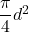d = Diamter = 10 cm

N = Number of turns = 60 turn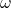= Angular velocity = 8 rad/s

Peak EMF is given by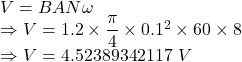Peak EMF is 4.52389342117 V

Time is given by (maximum)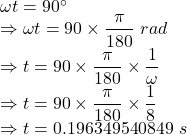Peak EMF is reached in 0.196349540849 s

Negative case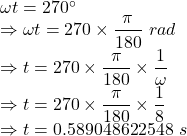The time taken is 0.589048622548 s

For output voltage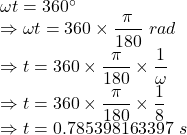Time taken is 0.785398163397 s